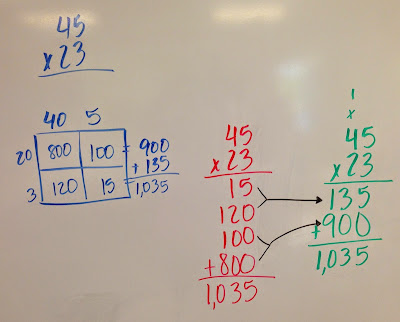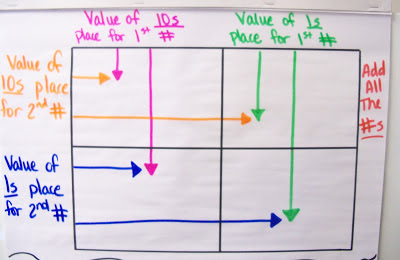## Friday, October 28, 2016

### Fraction Review and Intro to Improper Fractions

This week we took a brief look at fractions. We started by reviewing what we already know about fractions such as definitions and pictures.

After we reviewed, we looked at pieces of fractions and discussed what happens when the numerator and denominator are the same number. We looked at pictures of pizzas cut in half. Once I have two halves, I have one whole. Finally, we explored what happens when we have more than one whole. We discussed how we would name these improper fractions (we'll learn about mixed numbers a little later this year.) We named them on a number line, as well as in picture form.

## Monday, October 24, 2016

### Moving to the Standard Multiplication AlgorithmThe progression from matrix box to standard algorithm
This week we moved to the standard American algorithm for multiplication. Parents are always thrilled when we get to this, as this is the way they learned! We began by looking at our area model (in blue), and discuss what places were being multiplied during the process. The tens places of both numbers are multiplied together, then the tens of the first number is multiplied by the ones of the second number. Next the ones of the first number is multiplied by the tens of the second number. Finally, the ones of the first number is multiplied by the ones of the second number. Sounds confusing, right?! So we begin to discuss what this would look like vertically (in red). Then we discuss the traditional algorithm (in green), and make connections between all three strategies. This really helps your children understand the place value involved in the algorithm! Woohoo!

## Wednesday, October 19, 2016

### Multiplication Area Model

We finally got to begin 2-digit by 2-digit multiplication this week! I began by reviewing with them the area model we used when multiplying by 1 digit. Then the kids self-discovered (with my prompting and questioning) that they could create an area model for 2x2 multiplication. They referred to it as the "double-decker couch." Ha! The kids are absolutely loving the 2x2 area model!! They love it because it's super easy. I love is because it shows them the place value involved in 2x2 multiplication. They can actually see the process of multiplying the ones and tens of one number by the ones and tens of the second number. When they finally move to vertical multiplication, they'll actually understand why it works! There's nothing better than actually understanding WHY you do something!!!

The area model can actually be formatted to fit more than 2x2 multiplication. We played around with differently-sized numbers and made our matrix box fit those problems as well.Our class anchor chartClose-up of the box itselfAn example

## Friday, October 14, 2016

### Breaking Apart Multiplication

As we move to multiplying larger numbers, I like to guide them into the algorithm. We start by investigating the pattern when multiplying by multiples of 10. We look at the problems 2 x 3 = 6, 2 x 30 =60, and 2 x 300 = 600. We discuss what is the same/different about each problem, then we look at several other similar examples. Eventually the kids see the pattern of just "adding a zero" each time. Patterns are a great shortcut, but it's important the kids understand why they work.
Next we move to breaking apart larger multiplication problems, such as 18 x 5. We can break the 18 into 10 and 8 to help us multiply easier.

We practice this with many numbers, also looking at the array model for each. We slowly get to larger numbers like this one...
This will lead us into the multiplication algorithm next week.

## Monday, October 10, 2016

### Finding the Factors of 12

We begin our lesson on finding the factors of a number by making all the arrays we can think of for the number 12. The kids usually do a really good job finding them all. When I question if we've found them all, however, the kids start to second-guess themselves. This moves us into the discussion of what we're actually finding when we're making these arrays. I lead them to discover they're finding the factors (number that are multiplied to make a number) of 12.

But how can we keep our factors organized so we can make sure we've found all the factors? This leads us to making our T-Chart. We always start with 1 and the number itself. Then we move to the next number in the number line, 2. We know 2 is a factor because 12 is even, so we then find the partner for 2 which is 6. Then we move to 3, which has a partner of 4. The next number in the number line is 4, which we've already used, so we know we're done. Once numbers start to repeat, we've found all our factors. This lesson is followed by lots of practice finding the factors of many different numbers. As we're making our factor lists, we discuss the definition of prime, composite, and square numbers.

## Thursday, October 6, 2016

### Elapsed Time

Elapsed time can be a challenge for kiddos because they like to try to find the difference between two times by lining them up vertically and subtracting for the difference. In a whole-group discussion, we talk about why this won't always work. Our number system is base-10, which means when we reach 10 in a place, we must move it over to the place to the left. For example, when we get 10 ones, we turn it into 1 ten. We also borrow in groups of ten. Time does not follow these rules, so we cannot always successfully borrow or carry vertically.